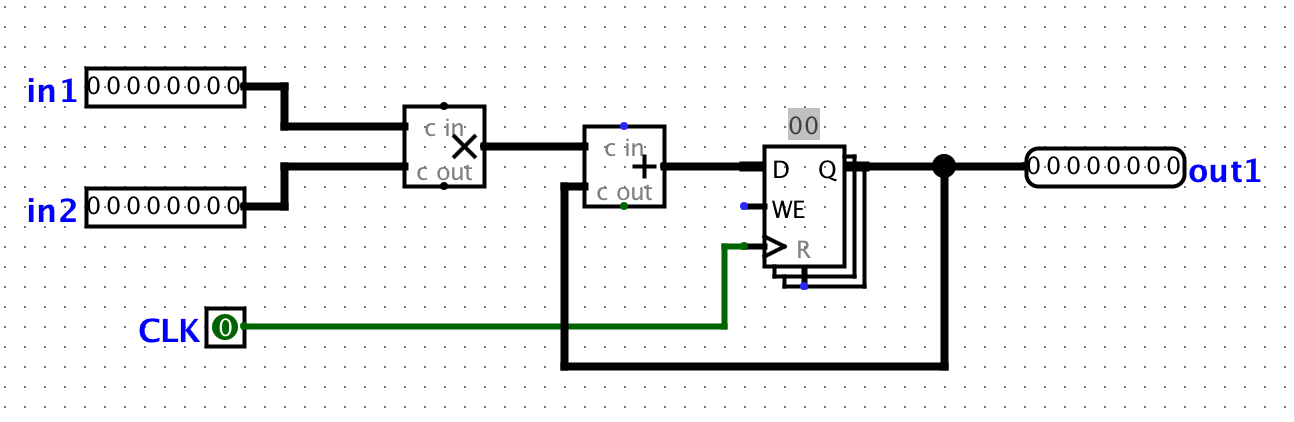## Lab 7

Computer Architecture I ShanghaiTech University
Lab 6 Lab 7 Lab 8

## Setup

Please use the Logisim provided in Lab 5.

## Exercises

### Exercise 1: Inefficiencies Everywhere

For this exercise, we can assume that registers initially carry the value zero. We will be using the lab file ex1.circ, which should have a subcircuit called non_pipelined which looks something like this:All this circuit does is take in two inputs, multiply them together, and then add the result to the current state value. For this circuit, let the propagation delay of an adder block be 45ns and the propagation delay of a multiplication block be 60ns. The register has a CLK-to-Q delay of 10ns, setup time of 10ns, and hold time of 5ns. Calculate the maximum clock rate at which this circuit can operate. Assume that both inputs come from clocked registers that receive their data from an outside source.

#### Checkoff

• Show your TA the calculations you performed to find the maximum clock rate (non-pipelined).

### Exercise 2: Pipe that Line

We want to improve the performance of this circuit and let it operate at a higher clock rate. In order to accomplish this, we want to have two stages in our pipeline: a multiplication stage and an addition stage, in that order.

In order to check that your pipelining still produces correct outputs, we will consider the outputs from the circuit "correct" if and only if it corresponds to the sequence of outputs the non-pipelined version would emit, bar some leadings zeroes. For example, if for some sequence of inputs, the non-pipelined version emits the sequence [3, 5, 1, 2, 4, …]. Then, the correct pipelined circuit might emit the sequence [0, 3, 5, 1, 2, 4, …] for the same sequence of inputs. You can check this by simulating the circuit (using the "Simulate" menu dropdown) and either ticking the clock manually or enabling continuous ticks.

In your ex1.circ file, the main circuit is set up to produce the output sequence [3, 5, 1, 2, 4, -1, 0, 0, …] from the non-pipelined version of the circuit. The ROM blocks should be initialized to the proper inputs, but if something goes wrong, select the ROM, click on "Contents", click "Open", then choose ROMdata.

Note that in order to pipeline the circuit, we need a register to hold the intermediate value of the computation between pipeline stages. This is a general theme with pipelines.